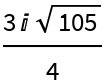# HeronFormula

Contributed by: Ed Pegg Jr

Given edge lengths of a triangle return the area

 ResourceFunction["HeronFormula"][{a,b,c}] gives the area of triangle with edge lengths (a,b,c).

## Examples

### Basic Examples

A 3-4-5 right triangle has area 6:

 In:=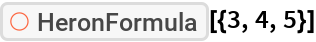Out=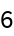A 13-14-15 triangle has area 84:

 In:=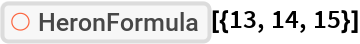Out=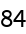### Properties and Relations

Degeneracy is not checked:

 In:=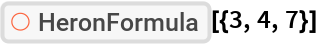Out=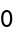In:=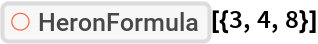Out=